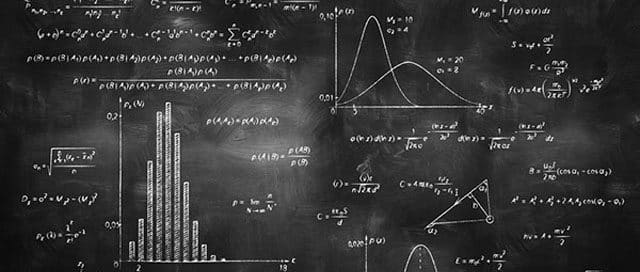# Semiconductors QuestionsQues: A P-type semiconductor can be obtained by adding
(a) Arsenic to pure silicon
(b) Gallium to pure silicon
(c) Antimony to pure germanium
(d) Phosphorous to pure germanium

Ans. (b)

Ques: When the electrical conductivity  of a semi-  conductor  is due to the breaking of its covalent bonds, then the semiconductor is said to be
(a) Donar
(b) Acceptor
(c) Intrinsic
(d) Extrinsic

Ans. (c)

Ques: Which statement is correct?
(a) N-type germanium is negatively charged and P-type germanium is positively charged
(b) Both N-type and P-type germanium are neutral
(c) N-type germanium is positively charged and P-type germanium is negatively charged
(d) Both N-type and P-type germanium are negatively charged

Ans. (b)

Ques: The impurity atoms which are mixed with pure silicon to make a P-type semiconductor are those of
(a) Phosphorus
(b) Boron
(c) Antimony
(d) Copper

Ans. (b)

Ques: At zero Kelvin a piece of germanium
(a) Becomes semiconductor
(b) Becomes good conductor
(d) Has maximum conductivity

Ans. (c)

Related: Human Health and Disease MCQs

Ques: In a good conductor the energy gap between the conduction band and the valence band is
(a) Infinite
(b) Wide
(c) Narrow
(d) Zero

Ans. (d)

Ques: A semiconductor is cooled from T1K to T2K. Its resistance
(a) Will decrease
(b) Will increase
(c) Will first decrease and then increase
(d) Will not change

Ans. (b)

Ques: The temperature coefficient of resistance of a semiconductor
(a) Is always positive
(b) Is always negative
(c) Is zero
(d) May be positive or negative or zero

Ans. (b)

Ques: An N-type and P-type silicon can be obtained by doping pure silicon with
(a) Arsenic and Phosphorous
(b) Indium and Aluminium
(c) Phosphorous and Indium
(d) Aluminium and Boron

Ans. (c)

Ques: For germanium crystal, the forbidden energy gap in joules is
(a) 1.12 x 10–19
(b) 1.76 x 10–19
(c) 1.6 x 10–19
(d) Zero

Ans. (a)

Ques: In a P-type semiconductor, germanium is doped with
(a) Boron
(b) Gallium
(c) Aluminium
(d) All of these

Ans. (d)

Related: photorespiration questions

Ques: At room temperature, a P-type semiconductor has
(a) Large number of holes and few electrons
(b) Large number of free electrons and few holes
(c) Equal number of free electrons and holes
(d) No electrons or holes

Ans. (a)

Ques: The process of adding impurities to the pure semiconductor is called
(a) Drouping
(b) Drooping
(c) Doping
(d) None of these

Ans. (c)

Ques: Which of the following has negative temperature coefficient of resistance?
(a) Copper
(b) Aluminium
(c) Iron
(d) Germanium

Ans. (d)

Ques: Which of the following statements is true for an N-type semi-conductor?
(a) The donor level lies closely below the bottom of the conduction band
(b) The donor level lies closely above the top of the valence band
(c) The donor level lies at the halfway mark of the forbidden energy gap
(d) None of above

Ans. (a)

Related: planck’s quantum theory quiz

Ques: In a P-type semi-conductor, germanium is dopped with
(a) Gallium
(b) Boron
(c) Aluminium
(d) All of these

Ans. (d)

Ques: The charge on a hole is equal to the charge of
(a) Zero
(b) Proton
(c) Neutron
(d) Electron

Ans. (b)

Ques: In extrinsic semiconductors
(a) The conduction band and valence band overlap
(b) The gap between conduction band and valence band is more than 16 eV
(c) The gap between conduction band and valence band is near about 1 eV
(d) The gap between conduction band and valence band will be 100 eV and more

Ans. (c)

Ques: The energy gap of silicon is 1.14 eV. The maximum wavelength at which silicon will begin absorbing energy is
(a) 10888 Å
(b) 1088.8 Å
(c) 108.88 Å
(d) 10.888 Å

Ans. (a)

Ques: The mobility of free electron is greater than that of free holes because
(a) The carry negative charge
(b) They are light
(c) They mutually collide less
(d) They require low energy to continue their motion

Ans. (d)

Ques: Doping of intrinsic semiconductor is done
(a) To neutralize charge carriers
(b) To increase the concentration of majority charge carriers
(c) To make it neutral before disposal
(d) To carry out further purification

Ans. (b)

Ques: To a germanium sample, traces of gallium are added as an impurity. The resultant sample would behave like
(a) A conductor
(b) A P-type semiconductor
(c) An N-type semiconductor
(d) An insulator

Ans. (b)